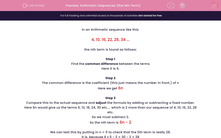# Arithmetic Sequences (the Nth Term)

In this worksheet, students find the Nth term in simple linear sequences.Key stage:  KS 2

Curriculum topic:   Maths and Numerical Reasoning

Curriculum subtopic:   Equations and Algebra

Difficulty level:#### Worksheet Overview

In an Arithmetic sequence like this:

4, 10, 16, 22, 28, 34 ...

the nth term is found as follows:

Step 1

Find the common difference between the terms

Here it is 6.

Step 2

The common difference is the coefficient (this just means the number in front.) of n

Here we get 6n

Step 3

Compare this to the actual sequence and adjust the formula by adding or subtracting a fixed number.

Here 6n would give us the terms 6, 12, 18, 24, 30 etc.... which is 2 more than our sequence of 4, 10, 16, 22, 28 etc.

So we must subtract 2.

So the nth term is 6n - 2

We can test this by putting in n = 5 to check that the 5th term is really 28.

It is, because 6 x 5 - 2 = 30 - 2 = 28

For this worksheet, It is important that you do not put spaces in your answer (For example, you would need to put in 5n-3 instead of 5n  -  3. If you put in spaces, the system will mark it as wrong.

### What is EdPlace?

We're your National Curriculum aligned online education content provider helping each child succeed in English, maths and science from year 1 to GCSE. With an EdPlace account you’ll be able to track and measure progress, helping each child achieve their best. We build confidence and attainment by personalising each child’s learning at a level that suits them.

Get started